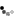# Higher-order Functions

### Passing an inline function as parameter

xquery version "3.0"; declare namespace ex="http://exist-db.org/xquery/ex"; declare function ex:apply(\$func, \$list) { for \$item in \$list return \$func(\$item) }; (: Create an inline function and assign it to \$f2 :) let \$f2 := function(\$a) { upper-case(\$a) } return ex:apply(\$f2, ("Hello", "world!"))### Using a named function reference

xquery version "3.0"; declare namespace ex="http://exist-db.org/xquery/ex"; declare function ex:apply(\$func as function(item()) as item()*, \$list) { for \$item in \$list return \$func(\$item) }; (: Use function reference literal to find function at compile time :) let \$fApply := ex:apply#2 return \$fApply(function(\$a) { upper-case(\$a) }, ("Hello", "world!"))### Using a dynamic function lookup

xquery version "3.0"; declare namespace ex="http://exist-db.org/xquery/ex2"; declare function ex:fold-left( \$f as function(item()*, item()) as item()*, \$zero as item()*, \$seq as item()*) as item()* { if (fn:empty(\$seq)) then \$zero else ex:fold-left(\$f, \$f(\$zero, \$seq), subsequence(\$seq, 2)) }; (: Function reference is resolved dynamically at runtime :) let \$foldLeft := function-lookup(xs:QName("ex:fold-left"), 3) return \$foldLeft(function(\$a, \$b) { \$a * \$b}, 1, 1 to 5)## Basic higher-order functions

The default function library provides a number of basic functions which take function items as arguments:

• for-each
• filter
• fold-left
• fold-right
• for-each-pair

### fn:for-each

for-each(1 to 5, function(\$a) { \$a * \$a })### fn:filter

fn:filter(1 to 10, function(\$a) {\$a mod 2 = 0})## Closures

### Inline function using variable from local context

xquery version "3.0"; declare function local:apply(\$names as xs:string*, \$f as function(xs:string) as xs:string) { for \$name in \$names return \$f(\$name) }; let \$string := "Hello " let \$f := function(\$name as xs:string) { (: \$string will be copied into the functions context :) \$string || \$name } return local:apply(("Hans", "Rudi"), \$f)## Partial function application

When passing functions as arguments, it is often useful to be able to set certain fixed parameters when creating the function reference. XQuery 3.0 allows this using ? as an argument placeholder.

### Multiply each item in a sequence with a base value

xquery version "3.0"; declare namespace ex="http://exist-db.org/xquery/ex"; declare function ex:multiply(\$base, \$number) { \$base * \$number }; let \$fMultiply := ex:multiply(10, ?) return for-each(1 to 10, \$fMultiply)